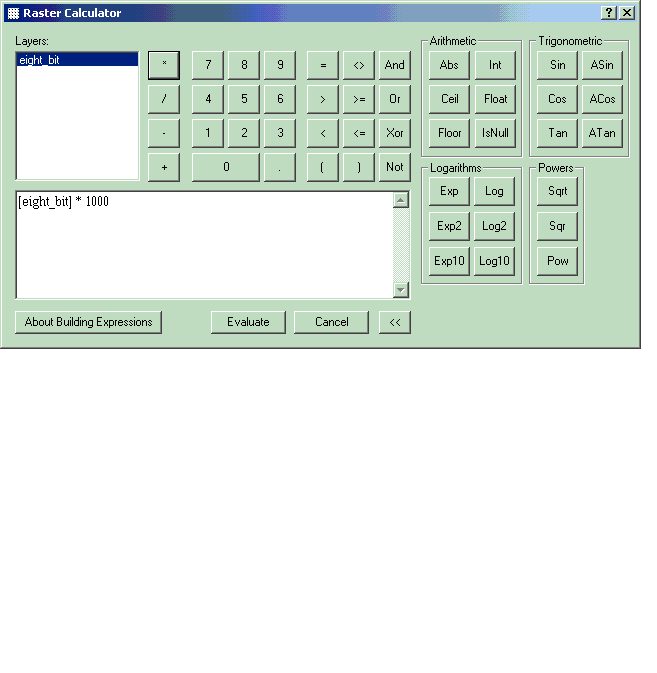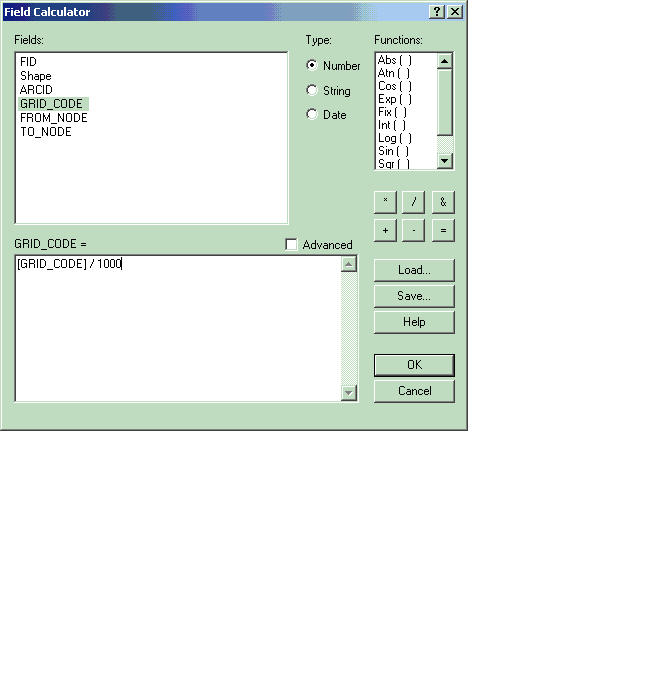ERROR

# Parameter: Value is outside the domain

## Error Message

When converting a floating point raster to a point, line, or polygon shapefile using ArcToolbox > Conversion Tools > From Raster, an error message displays:

"Parameter: Value is outside the domain."

## Cause

It is only possible to convert an integer raster to a shapefile. When converting an integer raster to a polygon, it rounds the raster's values to the nearest integer.

## Solution or Workaround

Convert a floating point raster to a shapefile while retaining the original raster values through the steps below:

`Note:Spatial Analyst is required.`

1. Multiply the image by how many decimal places are present using the Raster Calculator. For example, if the decimal ranges to the one thousandth place, multiply the image by 1000.
`1.  From the Spatial Analyst toolbar click Spatial Analyst dropdown list, and select Raster Calculator.2.  Double-click the image under 'Layers:' to add it to the dialog box below.3.  Select the multiplication button (*).4.  Type 1000.5.  Click Evaluate. 6.  A new raster called Calculation is added to the table of contents.`2. The new image, Calculation, is still a floating point raster. Convert this to an integer by going to ArcToolbox > Spatial Analyst Tools > Math > Int. Specify the Calculation raster as the Input raster, and then specify an output raster location and name.
3. Convert the integer raster to the shapefile geometry type of your choice using ArcToolbox > Conversion Tools > From Raster. Select either Raster to Point, Raster to Polygon, or Raster to Polyline.
4. The new shapefile is added to ArcMap. Start an edit session and open up the attribute table.
5. Right-click on the field GRID_CODE > Calculate Values.
6. Select GRID_CODE from the list of fields and divide this field by the same number as the raster was multiplied by in Step 1.The results are the original floating point raster values.

Article ID:000008672

Software:
• ArcMap 8 x
• ArcMap 9 x

Get help from ArcGIS experts

Contact technical support